# find the x-intercepts of the polynomial fuction

find the x-intercepts of the polynomial fuction.state weather the graph crosses the x-axis or touches the x-axis and turns around at each intercept.
x^5-30x^3+125x=0

x^5 - 30x^3 + 125x = 0 Factor out x x(x^4 - 30x^2 + 125) = 0 Factor the quadratic x(x^2 - 5)(x^2 - 25) = 0 three solutions x = 0 and x = +/-{{{sqrt(5)}}} and x = +/-{{{sqrt(25)}}} x = +/-5 : Each intercept crosses the x axis, as seen on the graph {{{ graph( 300, 200, -8, 8, -100, 100, x^5-30x^3+125x) }}}

#### Earn Coin

Coins can be redeemed for fabulous gifts.

Similar Homework Help Questions
• ### Find the x-intercepts of the polynomial function State whether the graph crosses the x-axis, or touches...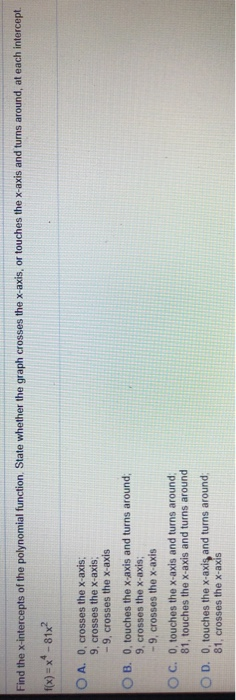Find the x-intercepts of the polynomial function State whether the graph crosses the x-axis, or touches the x-axis and turns around, at each intercept. f(x) = ** -81x? O A. O, crosses the x-axis: 9. crosses the x-axis: -9, crosses the x-axis OB. O, touches the x-axis and turns around; 9, crosses the x-axis; -9, crosses the x-axis OC. 0, touches the x-axis and turns around 81, touches the x-axis and turns around OD. O, touches the x-axis and turns...

• ### Question 12 of 25 > >> Question 12 1 points Save Answer Find the zeros for...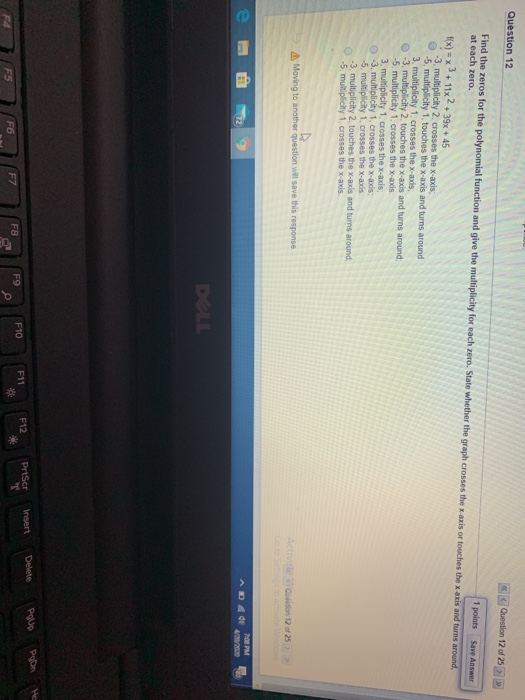Question 12 of 25 > >> Question 12 1 points Save Answer Find the zeros for the polynomial function and give the multiplicity for each zero. State whether the graph crosses the x-axis or touches the x-axis and turns around, at each zero. f(x) = x +11x2 + 3x + 45 3. multiplicity 2. crosses the x-axis -5 multiplicity 1. touches the x-axis and turns around 3. multiplicity 1. crosses the x-ads , multiplicity 2. touches the x-axis and turns...

• ### Find the zeros for the given polynomial function and give the multiplicity for each zero. State...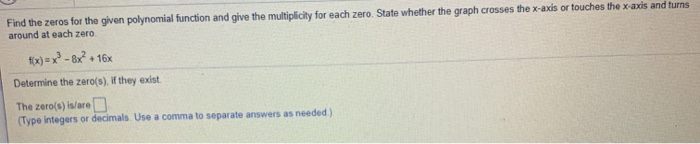Find the zeros for the given polynomial function and give the multiplicity for each zero. State whether the graph crosses the x-axis or touches the x-axis and turns around at each zero fix)=x2-8x? + + 16x Determine the zero(s). If they exist. The zero(s) is/are D (Type integers or decimals. Use a comma to separate answers as needed) Find the zeros for the given polynomial function and give the multiplicity for each zero. State whether the graph crosses the x-axis...

• ### Review for Sections 3.2 and 3.3: 1. [3 pts] Use synthetic division to divide x5 –...

Review for Sections 3.2 and 3.3: 1. [3 pts] Use synthetic division to divide x5 – x4 – 3x3 – 4x2 + 2x – 51 by x – 3. (Show algebraic work.) 2. [4 pts] Solve the equation x3 – 11x2 + 38x – 40 = 0 given that 4 is a zero of f (x) = x3 – 11x2 + 38x – 40. (Show algebraic work.) 3. [5 pts] Answer parts a. – c. below for the given polynomial...

• ### Answer parts a.-e. for the function shown below. #x-x3-4x2-x+4 a. Use the leading coefficient tes...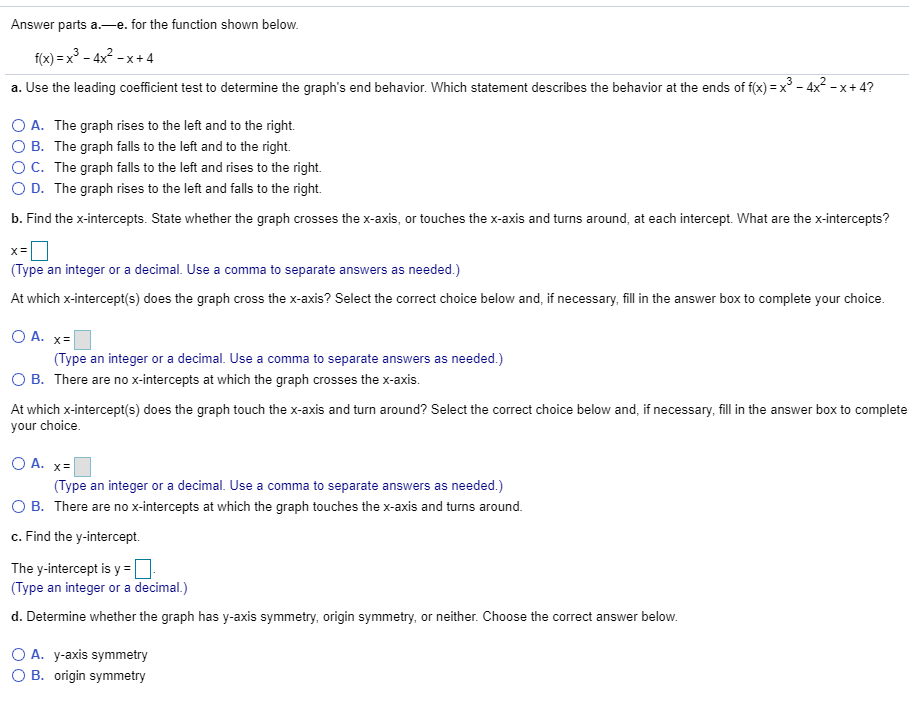Answer parts a.-e. for the function shown below. #x-x3-4x2-x+4 a. Use the leading coefficient test to determine the graph's end behavior. Which statement describes the behavior at the ends of f(x)=x"-4X-x+4? O A. The graph rises to the left and to the right. B. The graph falls to the left and to the right. ° C. The graph falls to the left and rises to the right. D. The graph rises to the left and falls to the right. b....

• ### Analyze the polynomial function f(x)=x2 + x2 - 20x. Complete parts (a) through (c). (a) Find...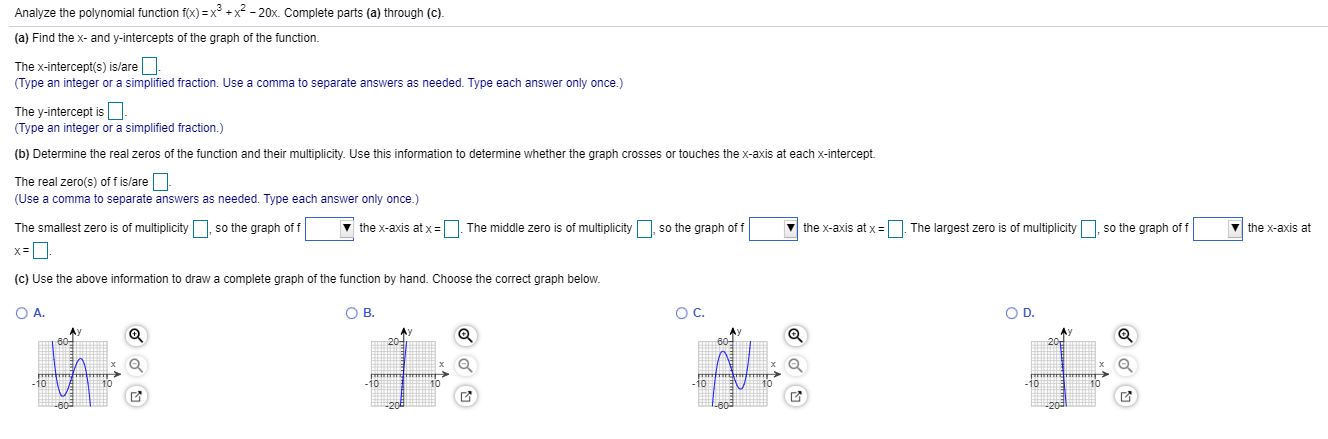Analyze the polynomial function f(x)=x2 + x2 - 20x. Complete parts (a) through (c). (a) Find the x- and y-intercepts of the graph of the function. The x-intercept(s) is/are - (Type an integer or a simplified fraction. Use a comma to separate answers as needed. Type each answer only once.) The y-intercept is - (Type an integer or a simplified fraction.) (b) Determine the real zeros of the function and their multiplicity. Use this information to determine whether the graph...

• ### For the polynomial, list each real zero and its multiplicity

For the polynomial, list each real zero and its multiplicity. Determine whether the graph crosses or touches the x-axis at each x -intercept. f(x) = 3(x + 6)(x + 5)4

• ### graph

determine the end behavior of the graph,find the zeros for each polynomial function,and give the multiplicity for each zero.state whether the graph crosses thex-axis,or touches the x-axis and turns around,at each zero.F(x)=3x(x+5)(x+2)^2

• ### 2.3 2.5

Find the zeros for the polynomial function and give the multiplicity for each zero. State whether the graph crosses the x-axis or touches the x-axis and turns around at each zero.f(x)= -2(x-9)(x+3)2

• ### for the polynomial, list each real zero and its multiplicity

for the polynomial, list each real zero and its multiplicity. determine whether the graph crosses or touches the x-axis at each x-intercept. f(x)=1/2x^4(x^2-5)(x+1)